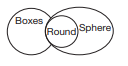# Logical Reasoning - Statement and Conclusion

>>>>>>>>Statement and Conclusion

In each of the questions below, there are two statements followed by three to four conclusions numbered as I, II, III, and IV. You have to take the given statements to be true even if they seem to be at variance with commonly known facts and then decide which of the given conclusions logically follow from the given statements.

• A

Only I, III, and IV follows.• B

Only II and III follows.• C

All conclusions follow• D

None of the conclusions follow.• Option : C
• Explanation : Only three terms (magic, women, and crazy). No alignment is required as they are in the standard format.
Now A + A = A.
Conclusion: All magic is crazy. So statement I is correct. Its immediate inference is ‘Some crazy are magic’. It means the third statement is true.
The converse of second statement ‘All women are crazy’ is ‘Some crazy are women’. So fourth conclusion is also true. Thus, option (c) is the answer.

• A

No tiger is a mammal.• B

No mammals are tigers.• C

Cats are tigers.• D

None of the above• Option : D
• Explanation : There are three terms. After alignment,
Some mammals are cats.
No cats are tigers.
If we look at conclusions directly, the term ‘male teacher’ in the first conclusion and ‘women teachers’ and ‘present’ in the second conclusion are not mentioned in statements. So, option (d) is the answer.

• A

I, II and III follows.• B

II, III and IV follows.• C

I, III and IV follows.• D

I, II and IV follows.• Option : A
• Explanation : I + A = I. The conclusion is some boxes are spheres which is I. This can be converted to some spheres are boxes, which is II. Conclusion III follows after converting all rounds are spheres. So, option (a) is the answer. Venn diagram solution:• A

I and III follows.• B

Only I follows.• C

Either I or II follows.• D

Either III or IV and I follows.• Option : B
• Explanation : They are in desired format A to B and B to C.
All books are clocks (A-type)
Some clocks are chips (I-type)
A + I = No conclusion.
Now, we can check first statement after immediate inference—Some clocks are books.
Second statement after immediate inference—Some chips are clocks.
So only conclusion I follows. Thus, option (b) is the answer.

• A

Only I and III follows.• B

Only II and IV follows.• C

All follow• D

None followsRelated Quiz.
Statement and Conclusion